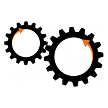# Unit Pre-Processing and Adaptive Plots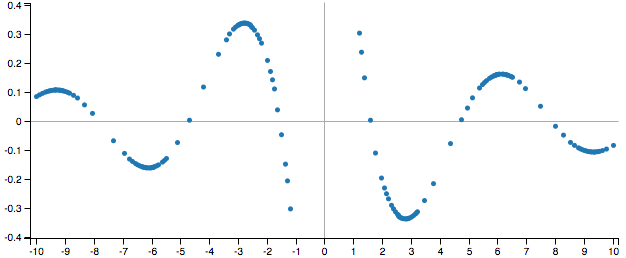Noticed speed improvements in Swift Calcs plots and solvers recently? It's not your imagination: Quietly slipped in to a weekly production release two weeks ago was an update to our mathematics engine that increases calculation speeds up to 100x, depending on the calculation.

The secret to the bump? Well, its actually two:

## Unit pre-processing

Working with units is a huge benefit of performing calculations with Swift Calcs, but the downside is that the inclusion of unit operators adds considerable overhead to each calculation. Units of the same type have to be converted during operations, checks have to be made to ensure unit consistency, and unit simplifications have to be done to remove canceled dimensions.

For most calculations, this added overhead is hardly noticed, but for operations that require continual re-evaluation of a function or expression, such as solvers and plots, this added overhead turns in to a real drag.

In these situations, however, we don't really need to do all the extra unit calculations on each and every iteration. We really need to do it once, up-front, to check for unit collisions or inconsistencies. Once we know the units are valid, we can simplify the expression by converting all units to mksa equivalents and then stripping them out.

In this way, we reduce the unit calculation overhead considerably by removing it from internal iterative routines. When we get our results, we simply re-apply the output unit to the answer. The results of this approach are dramatic...take a look at measured computation times for some functional tests before and after the change: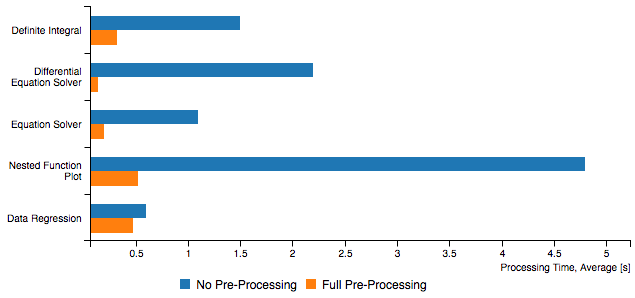For some calculations involving heavily nested functions with lots of unit calculations, this approach increased computation speed nearly 100x! And best of all, it works in the vast majority of situations...but when it doesn't (for example, when non-absolute temperature scales are utilized...ugh offsets!), we can simply revert to the previous method to perform the calculation.

When it comes to plots, however, we have more to share: Prior to last week's release, Swift Calcs used a plotting algorithm that is best described as brute-force. We would take a plot window, determine the number of pixels along the x-axis, and calculate the corresponding y value for each location. That equals 590 calculations for each function plotted. Consider y=x2 below using our old implementation...the number of sampled points is so large you can't really discern one point from the next: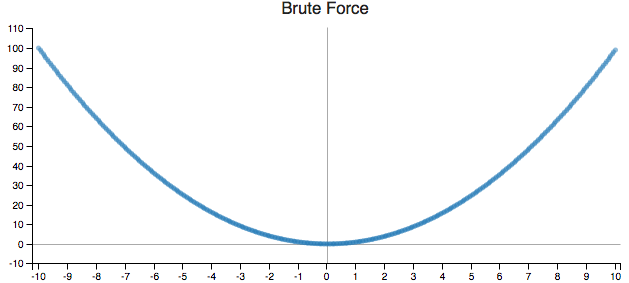This is overkill. Most functions don't need every pixel to be calculated to produce a visually accurate line. To reduce computational load, our new routine focuses on areas of high-curvature. We use an adaptive sampling routine that starts with a certain number of points (we use 20) roughly evenly spaced throughout the plot domain (not exactly even as we apply a bit of randomness to each point...if we didn't, we could get tripped up by periodic functions that have the exact same frequency as our sample points).

Next, we take a look at each sampled point and its two neighbors. If the angle formed by the three points is between 170 and 190 degrees, we have minimal curvature and no further refinement is needed (Tangent: The angle calculated is the perceived angle when plotted on the screen, as opposed to the true angle between the vectors. So its value depends on both the x-axis and y-axis limits, as well as the plot aspect ratio and use of log-plots). If we're outside these bounds, we calculate another point and at it to our sample, and again run the curvature comparison.

In this way, we continue to refine the plot until all sampled points are within the curvature bounds, or we've reached the resolution of the screen. Take a look at y=x2 plotted using our new method: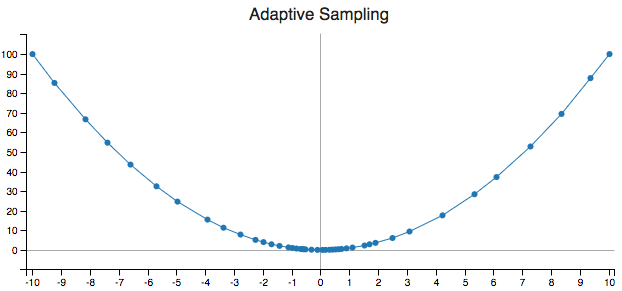And here are the two plots from before and after, with the sampling points removed to only show the functional form: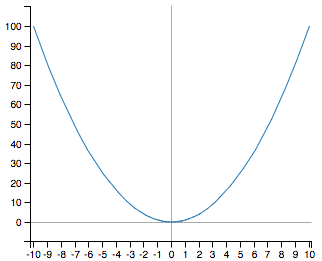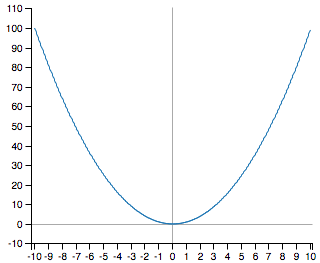The plots are visually indistinguishable (can you tell which is which?), but the number of calculations (and therefore the time) to produce the one on the left is over 10 times less.

Most functions we tested end up using somewhere between 60 to 120 points, representing speed improvements of 3-10x from our previous brute force method. Combined with the unit pre-processing above, we're seeing speed improvements of between 10-100x...plots now often render in fractions of a second!

## And added bonus: Dynamic Range

A nice benefit to the inclusion of the adaptive sampling algorithm is that a dynamic range solver comes along for free.

What is dynamic range? This is the idea that when producing a function plot where the y-axis bounds we're not set to specific values, the plotting engine should return appropriate y-axis bounds that produce a visually useful plot. For most functions, this range is simply the minimum and maximum y-values observed within the plot domain. However for plots with asymptotes that approach infinite values, the asymptote spike leads to an extremely large maximum or minimum value that create a visually useless plot.

Our calculation of the visual angle for the adaptive sampling technique, however, requires us to 'know' the y-axis range to determine the angle. Using this information, we can apply a simple test: Every time we add a new point in the sampling routine, if the new point is outside the currently known y-axis bounds, we consider the point to be 'outside' the preferred range if it would cause the value of: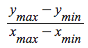to increase by a factor of 8 times or more. Otherwise, we simply increase our known y-axis bounds. This helps us ignore asymptotes when a plot blows up and focus on the portion of the function of interest. Take a look at the plot for y=cos(x)/x from before and after this change: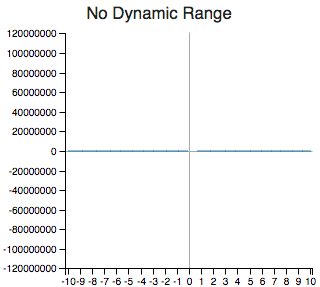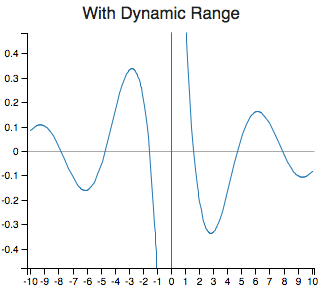Clearly the new plot is more useful than the old.